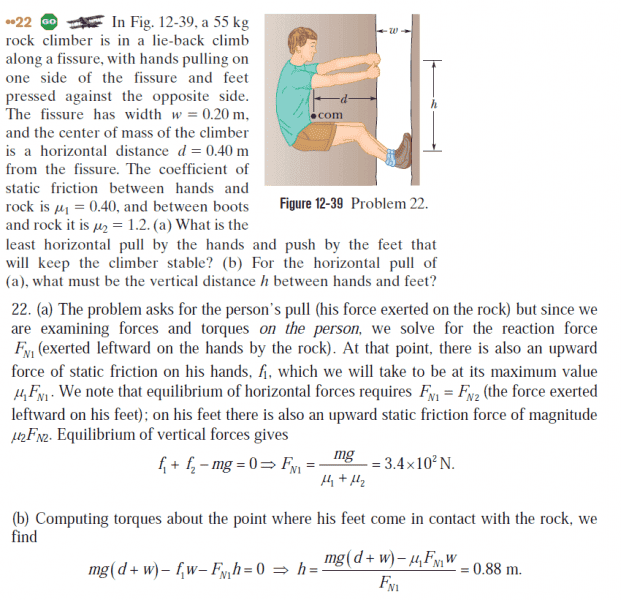# Static Equilibrium: Homework Questions

• Speedking96
In summary, the conversation discusses the concept of torque and rotational dynamics, specifically in relation to a problem involving normal forces and pivot points. The first part addresses the question of whether there should be a separate normal force for each hand in part a of the problem, and why the torque for Fn1h is not equal to zero in part b. The conversation then delves into the concept of pivot points and how they are used in calculations, with a reference to problems involving beams between two supports. The conversation ends with a suggestion to look at a specific resource for a better understanding of the topic and a question about the person's familiarity with levers.

## Homework Statement

I have a question about the following question:Firstly, shouldn't there be a normal force for each hand (part a), but here they treat it as one normal force.

Also in part b, why isn't the torque for Fn1h = 0? Since that is the pivot point, isn't the moment arm zero?

Firstly, shouldn't there be a normal force for each hand (part a), but here they treat it as one normal force.
How is the result different if you give each hand a separate normal force?

Also in part b, why isn't the torque for Fn1h = 0? Since that is the pivot point, isn't the moment arm zero?
##F_{N1} \neq 0## and ##h\neq 0## therefore ##F_{N1}h \neq 0## ... take a closer look: what are they using for the pivot point in that calculation?

Wow. The pivot point is the location of the foot and N1 is for the hands. I see. Thank you.

Well done. Remember all those horizontal mean static problems - beam between two supports?
Remember how you'd do a sum-of-torques for each support as the pivot?

I actually don't know what you're referring to as I am learning torque and rotational dynamics on my own (on my final exam tomorrow and my teacher rushed everything on rotation ) - I don't think I've come across a problem like that

Have a look at:
http://www.pstcc.edu/departments/natural_behavioral_sciences/Web%20Physics/Experiment%2002-1320.htm
... it counts as essential physics.

Have you done levers?